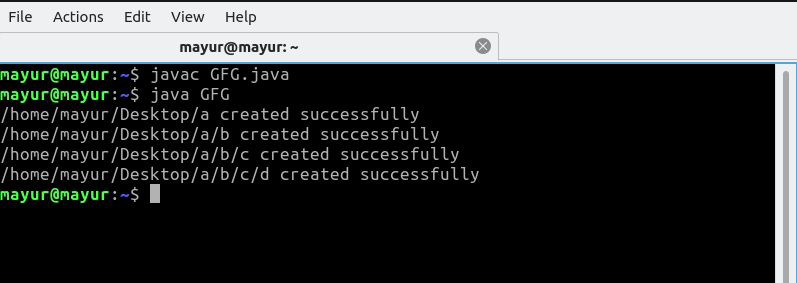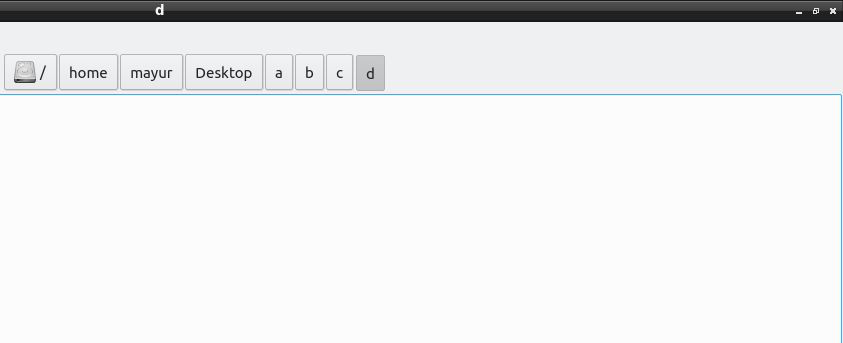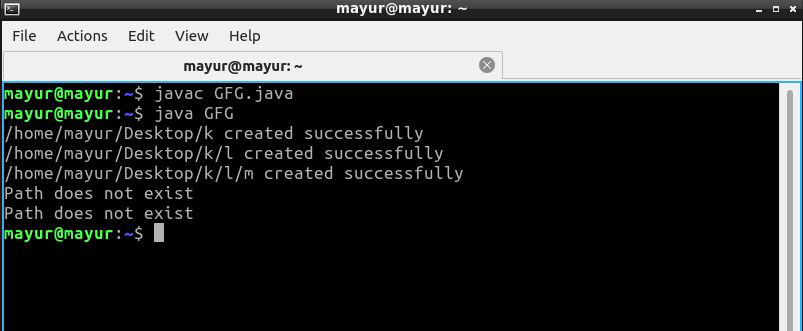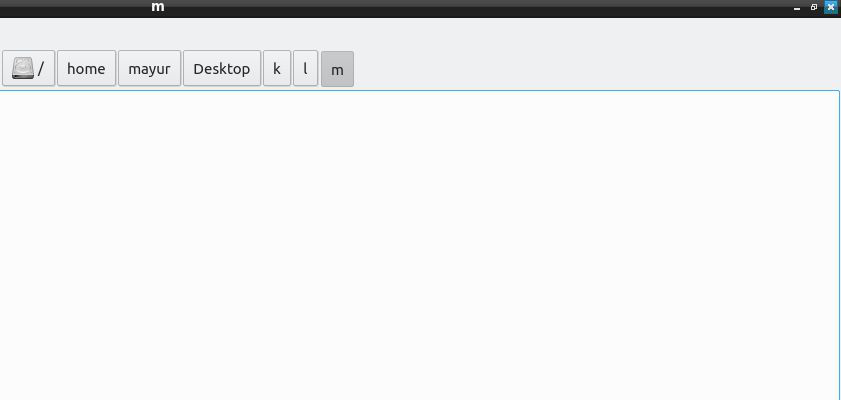Open in App
Not now

# Java Program to Create Directories Recursively

• Last Updated : 12 May, 2022

A directory/folder is a File System used in computing that acts as a named memory location for storing related files or even subfolders. This allows better management of files and folders and is premised on the concept of real-world folders used for storing files. This system is the implementation of compartmentalization of the memory and makes the working space more organized. The Directory File System allows hierarchical arrangement as well as nesting of directories inside other directories.

Recursion is the process through which a function calls itself. It is a very useful approach to break down complex problems into smaller subparts.

Approaches:

1. Using the mkdir() method
2. Using the createDirectory() method of the java.nio package

### 1. Using the mkdir() Method

The first approach is to import the java.io.File class and define a method named file() which internally makes use of the mkdir() function to recursively create directories. The algorithm used inside the file() method is described below.

Algorithm:

1. Create the file() method with the return type as void.
2. This method takes three parameters :
• String md which stands for Main Directory.
• String path which stands for the directory structure to be made where each character means a new directory
• Int depth represents the number of directories to be made.
3. Declare the terminating condition as if (depth == 0) return.
4. Decrement the depth for each recursive call.
5. Check if the path string is 0 in length and display the message accordingly.
6. Append the md with the first character of the path string and remove the first character from the path string for each recursive call.
7. Create an object of the File class with md as a parameter.
8. Check if the directory already exists using the exists() method and display the message.
9. Else create the directory using the mkdir()method.
10. Make the recursive call.

Implementation: Below is the implementation of the above program.

## Java

 `// Java Program to Create Directories Recursively`   `// Importing required classes` `import` `java.io.File;`   `// Main class` `class` `GFG {`   `    ``// Method` `    ``// To create directories` `    ``static` `void` `file(String md, String path, ``int` `depth)` `    ``{`   `        ``// md stores the starting path each character in` `        ``// path represents new directory depth stores` `        ``// the number of directories to be created` `        ``// terminating condition`   `        ``if` `(depth == ``0``)` `            ``return``;`   `        ``// Decrementing the depth by 1` `        ``depth -= ``1``;`   `        ``// Checking if the path exists` `        ``if` `(path.length() == ``0``)` `            ``System.out.println(``"Path does not exist"``);`   `        ``// execute if the path has more directories` `        ``else` `{`   `            ``// appending the next directory` `            ``// would be md = md + "\\" +` `            ``// path.charAt(0) for windows` `            ``md = md + ``"/"` `+ path.charAt(``0``);`   `            ``// removing the first character` `            ``// from path string` `            ``path = path.substring(``1``);`   `            ``// creating File object` `            ``File f = ``new` `File(md);`   `            ``// if the directory already exists` `            ``if` `(f.exists()) {` `                ``System.out.println(``"The Directory "` `                                   ``+ ``"already exists"``);` `            ``}` `            ``else` `{`   `                ``// creating the directory` `                ``boolean` `val = f.mkdir();` `                ``if` `(val)` `                    ``System.out.println(md + ``" created"` `                                       ``+ ``" successfully"``);` `                ``else` `                    ``System.out.println(` `                        ``"Unable to "` `                        ``+ ``"create Directory"``);` `            ``}` `        ``}`   `        ``// recursive call` `        ``file(md, path, depth);` `    ``}`   `    ``// Driver method` `    ``public` `static` `void` `main(String[] args)` `    ``{`   `        ``// creating class object` `        ``GFG ob = ``new` `GFG();`   `        ``// path for windows -> "C:\\Users\\` `        ``// harshit\\Desktop"` `        ``ob.file(``"/home/mayur/Desktop"``, ``"abcd"``, ``4``);` `    ``}` `}`

Output:### 2. Using thecreateDirectory()method of thejava.nio package

This approach makes use of java.nio package to implement the code. We deploy the createDirectories() method here to create new directories. We also make use of the try-catch block to catch IO Errors. The Algorithm can be found below.

Algorithm:

1. Repeat steps through 1-6 as mentioned in the algorithm in approach 1.
2. Now, convert the string md to Path Instance using Path.gets() method.
3. Again, check if the Directory already exists using the exists() method.
4. If the Directory is not present, open a try-catch block, and create a new directory using createDirectories() method.
5. Else, display that the Directory already exists.
6. Make the recursive call

Below is the implementation of the above program.

## Java

 `// Java code to create directories recursively`   `// importing the packages` `import` `java.nio.file.Paths;` `import` `java.nio.file.Path;` `import` `java.nio.file.Files;` `import` `java.io.IOException;`   `class` `GFG {`   `    ``// defining the recursive method` `    ``static` `void` `file(String md, String path, ``int` `depth)` `    ``{`   `        ``// base case` `        ``if` `(depth == ``0``)` `            ``return``;`   `        ``// decrement the depth` `        ``depth -= ``1``;`   `        ``// check if the path is empty` `        ``if` `(path.length() == ``0``)` `            ``System.out.println(``"Path does not exist"``);` `        ``else` `{`   `            ``// appending the first character from` `            ``// path string` `            ``md = md + ``"/"` `+ path.charAt(``0``);`   `            ``// removing the first character from` `            ``// path string` `            ``path = path.substring(``1``);`   `            ``// creating the path instance from` `            ``// path string` `            ``Path p = Paths.get(md);`   `            ``// if the directory already exists` `            ``if` `(!Files.exists(p)) {` `                ``try` `{`   `                    ``// creating directory` `                    ``Files.createDirectories(p);` `                    ``System.out.println(md + ``" created"` `                                       ``+ ``" successfully"``);` `                ``}` `                ``catch` `(IOException err) {` `                    ``err.printStackTrace();` `                ``}` `            ``}` `            ``else` `                ``System.out.println(``"The directory "` `                                   ``+ ``"already exists"``);` `        ``}`   `        ``// recursive call` `        ``file(md, path, depth);` `    ``}`   `    ``// Driver Code` `    ``public` `static` `void` `main(String[] args)` `    ``{`   `        ``// creating the object of the class` `        ``GFG ob = ``new` `GFG();`   `        ``// md would be -> "C:\\Users\\harshit\\` `        ``// Desktop for windows` `        ``ob.file(``"/home/mayur/Desktop"``, ``"klm"``, ``5``);` `    ``}` `}`

Output:My Personal Notes arrow_drop_up
Related Articles